# Play with the encoders

In the second guide, we'll experiment with another type of input: encoders.

We'll go through how to code the pre-drawn circle on the Track matrix so that you may adjust its width with the encoders.

Make a new variable called "width" first.

We'll set the value of width at 8 at the beginning.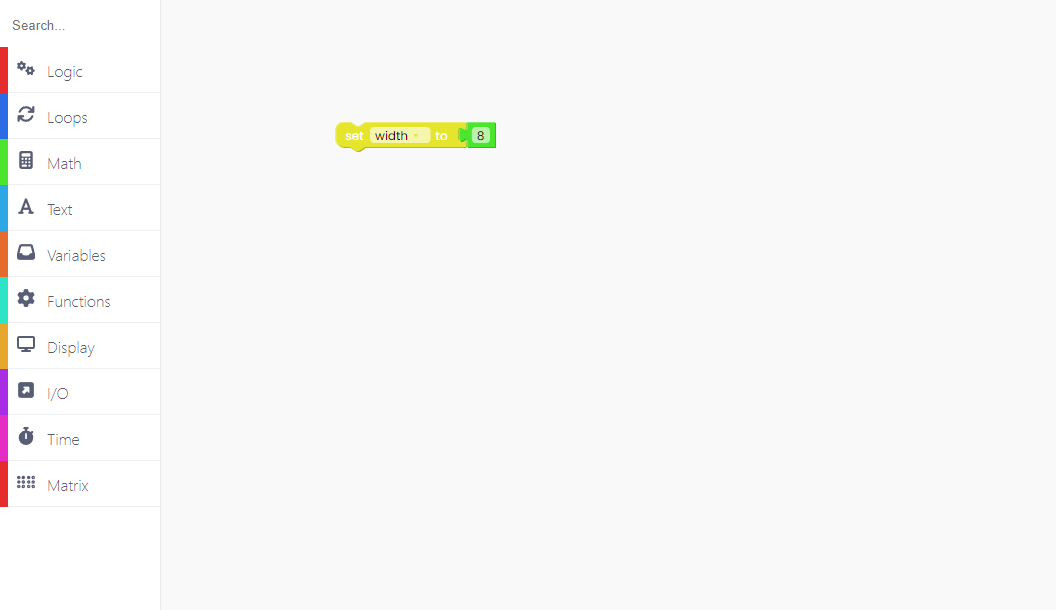Now we'll write code to see what happens to this variable when we move the left encoder to the right or left

You'll need I/O blocks, similar to the ones you used in the last example.We want the circle to narrow as we move it to the left.

As a result, we'll set the value of the variable "width" to the difference between the variable's value and 1.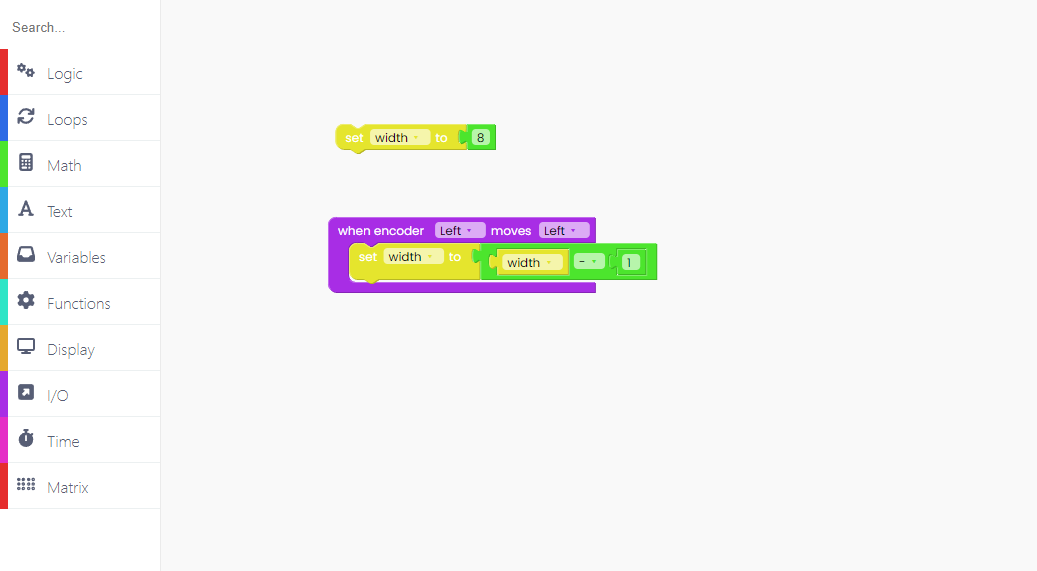However, because that is the maximum, we must limit the number to between 0 and 16.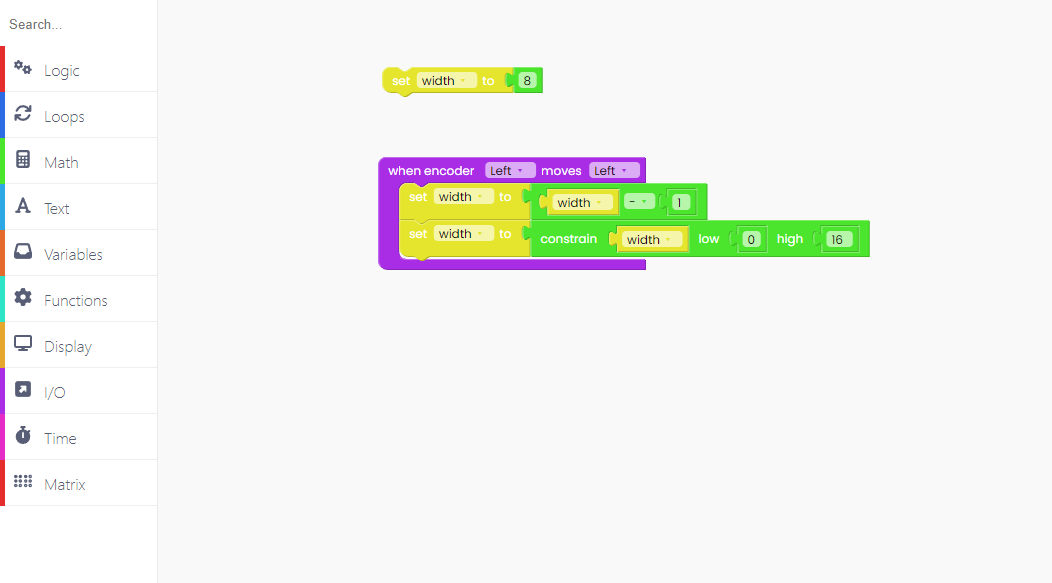Let's do this for turning the Left encoder to the right..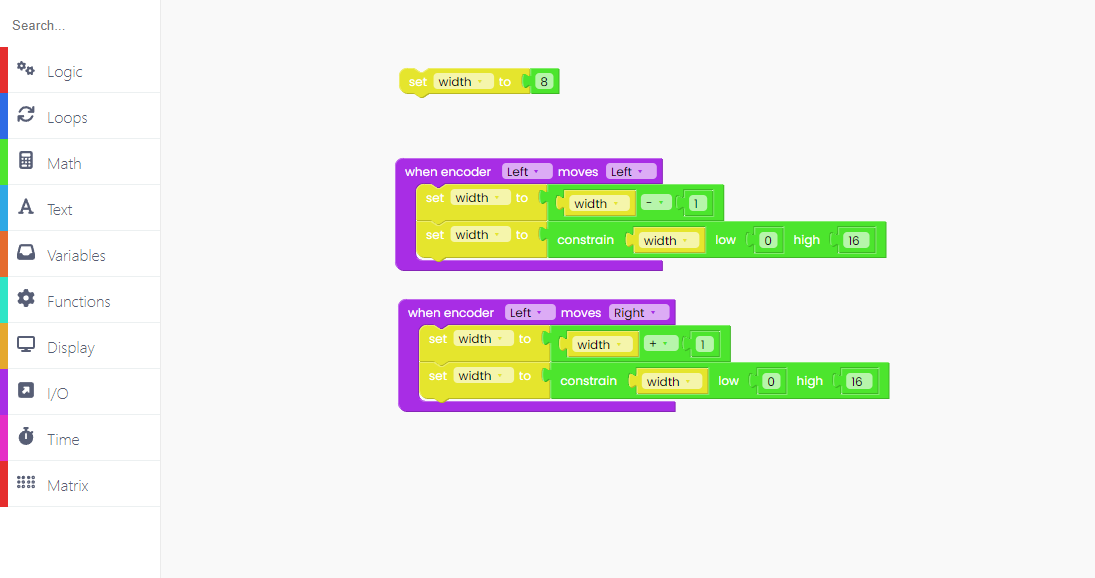This time, we'll increase the width value by one.

Now is the time to draw what will be happening on the matrix.

As mentioned before, we want to draw the circle.

Firstly, find the "loop forever" block inside the "Loops" block section.To begin, we must turn off all of the LEDs.

Find the "fill matrix frame with 0" block to do so.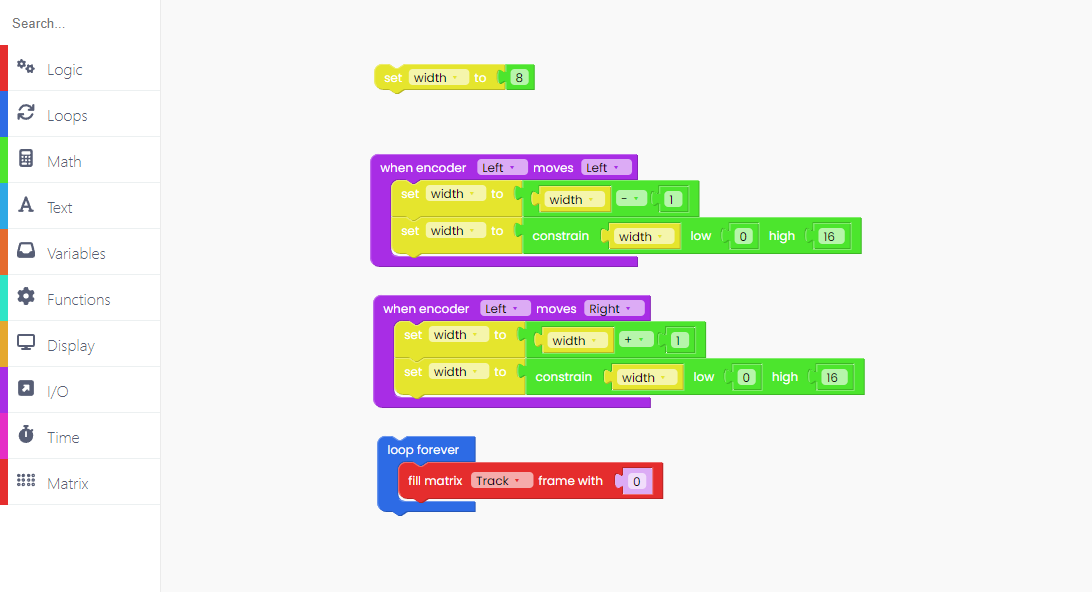With this block, we reduced the intensity of all LEDs on the Track matrix to 0 - turning them off.

Now, in the "Matrix" block section, locate this large block and place it into the "Loop forever" block.We use the variable "width" for the width, which means that it will be 8 at first but can change based on how the encoders are turned.

The height is set to 5, the x and y coordinates are set to 0, and the LED intensity is set to 100.

As we saw in the last example, you must include the "push frame to matrix" block every time you draw something on the matrix.

You must also remember the "scan encoders" block because you are working with them.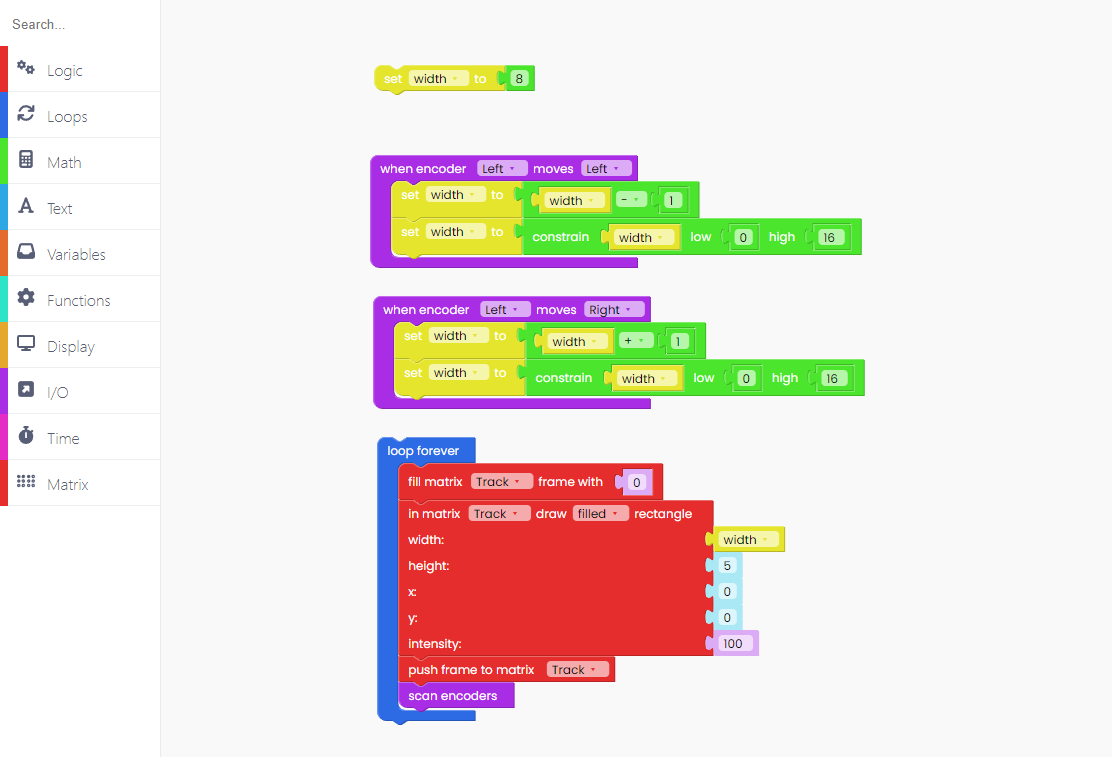Start turning the encoders after clicking the Run button